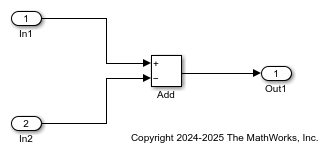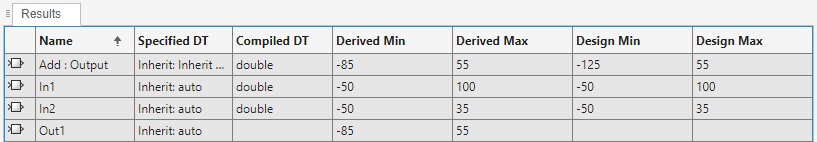Derive Ranges Using Design Ranges

This example shows how the range analysis narrows the derived range for the Outport block. This range is based on the range derived for the Add block using the design ranges specified on the two Inport blocks and the design range specified for the Add block.

Open the Model and View Design Ranges

Open the model. At the MATLAB command line, enter:

open_system('ex_derived_min_max_1')Update the diagram to display the specified design minimum and maximum values for each block.

• In1 design range is [-50..100].

• In2 design range is [-50..35].

• Add block design range is [-125..55].

Derive Ranges

1. From the Simulink® Apps tab, select Fixed-Point Tool.

2. In the Fixed-Point Tool, under New workflow, select Iterative Fixed-Point Conversion.

3. Under System Under Design (SUD), select ex_derived_min_max_1 as the system you want to convert.

4. Under Range Collection Mode, select .

5. Click the button.

To calculate the derived range at the Add block input, the software uses the design minimum and maximum values specified for the Inport blocks, [-50..100] and [-50..35]. The derived range at the Add block input is [-85..150].

When the analysis is complete, the Fixed-Point Tool displays the derived and design minimum and maximum values for the blocks in the selected system in the spreadsheet.• The derived range for the Add block output signal is narrowed to [-85..55]. This derived range is the intersection of the range derived from the block inputs, [-85..150], and the design minimum and maximum values specified for the block output, [-125..55].

Note

The accumulator in the Add block does not participate in derived range analysis. Ranges are derived only for block output signals.

• The derived range for the Outport block Out1 is [-85..55], the same as the Add block output.

Tip

To display design ranges in your model, in the tab, select .

Fixed-Point Designer DocumentationGet trial now